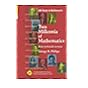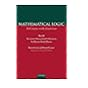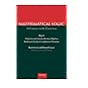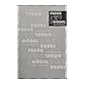Normal view MARC view ISBD view

# A course of higher mathematics. Vol. II: Advanced calculusNo cover image available No cover image available510.08 CAR Algebra and tiling - homomorphisms in the service of geometry. 510.08 CAR Knot theory. 510.09 PHI Two millennia of mathematics : from Archimedes to Gauss 511 SMI A course of higher mathematics. Vol. II: Advanced calculus 511.2 COR Mathematical logic : a course with exercises. 511.2 COR Mathematical logic : a course with exercises 511.2 HEI From Frege to Gödel : a source book in mathematical logic, 1879-1931